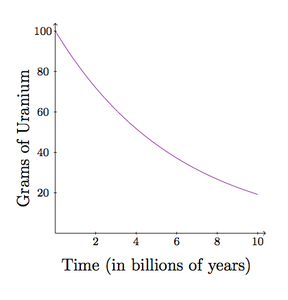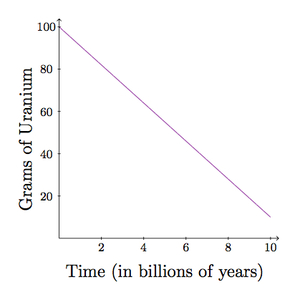# Uranium 238

Alignments to Content Standards: A-CED.A.2

Uranium 238 is a radioactive material with many applications in nuclear technology. It decays exponentially with a half-life of about 4.5 billion years.

1. Write an equation expressing how much Uranium 238 remains after $t$ years assuming the initial sample size is 100 grams.
2. For each graph below, decide whether or not the graph could represent the equation that you found in part (a):
1.2.3.## IM Commentary

The goal of this task is to represent an exponential relationship by an equation and identify, using knowledge of the context and the structure of the equation, possible graphs for the equation. The teacher may wish to provide more possible graphs to highlight different aspects of the exponential equation. In addition, the teacher will need to make sure that students understand the context, that is that they understand that the half-life is the amount of time it takes for half of the Uranium 238 to decay. For much further information about the radioactive decay cycle and technological uses of Uranium 238, see http://en.wikipedia.org/wiki/Uranium-238.

## Solution

1. We will let $f(t)$ be the amount of uranium 238 remaining from the sample (measured in grams) after $t$ years. We use billions of years as units of time and we let $t=0$ represent the initial moment when there are 100 grams of uranium 238.  Since the uranium decays exponentially, the function $f$ has the form $f(t) = ae^{-ct}$ where $a$ and $c$ are positive real numbers. Since we start with 100 grams of uranium, $f(0) = 100$. We also have $f(0) = ae^0=a$ so this tells us that $a = 100$. We also know that $f(4.5) = 50$ because after 4.5 billion years half of the original uranium sample will remain. This means $$100e^{-4.5c} = 50$$ and so $e^{-4.5c} = \frac{1}{2}$. Taking natural logarithms of both sides we find $c = \frac{\ln{\frac{1}{2}}}{-4.5} \approx 0.15$. So our model for the amount of remaining uranium in the sample is $f(t) = 100e^{-0.15t}$.

2. The first graph shows the amount of uranium in the sample decreasing over time which is accurate. It also shows that after a little over 4 billion years there are only 50 grams of uranium remaining. It has the shape of a decreasing exponential graph and we can also verify that after 9 billion years (two half-lives) there are about 25 grams remaining. So the first graph could be accurate.

The second graph is linear. Although it shows the correct values at 0 years and after 4.5 billion years, this is incorrect. The linear function will very quickly become zero and already takes too small of a value at 9 billion years.

The third graph is interesting. In fact it is the same as the first graph except that the variables (amount of uranium and time) have been switched. Thus this could also be accurate. We are used to plotting time as the independent variable but in a situation of exponential decay, either variable can be viewed as independent. The last choice may feel odd but it is an accurate depiction of the relationship between time and the amount of remaining uranium.